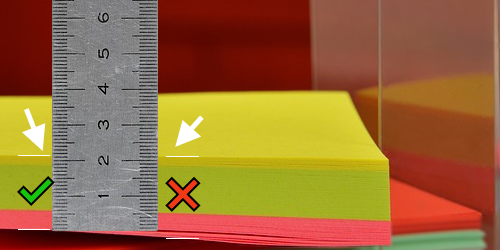# Paper Thickness Calculator

Created by Kenneth Alambra
Reviewed by Dominik Czernia, PhD candidate and Jack Bowater
Last updated: Mar 10, 2022

This paper thickness calculator will help you find the thickness of a paper sheet with just the regular size measuring tools we have at home or at the office.

Knowing how to calculate the thickness of a paper, whether you are going back to school or working at an office, is handy especially if you do a lot of printing and you only have a basic printer to use. Some printers can only print on a specific thickness of paper so knowing how thick a piece of paper is could help you save time and the struggle of clearing up a paper jammed printer.

## How thick is a piece of paper?

During the manufacturing process of paper, the pulp from wood or recycled paper goes through many passes between cylindrical rollers to join together their fibers to form paper and squeeze out its water content.

At some point in the process, the paper passes between two precisely spaced rollers that determine the paper's final thickness. For the rest of the coating and finishing process of papermaking, this thickness of the paper is maintained until it is cut into its final sizes.

Paper thickness range from the thinnest 0.02 mm papers up to around very thick 1.20 mm cardstock. Common office paper ranges from 0.05 mm to 0.10 mm in thickness.

We can use specialized precision tools, like micrometers and calipers, to easily measure how thick a piece of paper is. However, these tools are not commonly available at home. In the next section of this text, let us discuss how to calculate the thickness of a sheet of paper with only the tools we have at home.

## How to calculate the thickness of a paper sheet?

Measuring very thin or tiny objects at home usually requires tools we may not even have. But worry no more, because we can easily determine the thickness of paper using a ruler or any similar tool you have at home together with some simple calculations.

To determine the thickness of a paper using a ruler, take some pieces of paper from the same pack and stack them up to a thickness you can measure with your ruler. Make sure to measure the part where the paper is neatly stacked and compressed together, as shown below:After measuring the thickness of the stack, divide this measurement by the number of paper sheets you have in the stack to obtain the paper thickness. And that's it! 😊

Let us consider an example to understand this even further.

## Sample calculation of paper thickness

Let's say you measure a ream of paper to be 2 inches thick (about 5.08 cm). Since a ream of paper contains 500 sheets of paper, we divide 2 inches by 500. This will then give us a value of 0.004 inches (about 0.0102 cm), which is the thickness of a single piece of paper.

For values as small as that number, we can use the caliper unit of measure. In a caliper, we express the thickness of paper in points (pt) where 1 pt is equal to a thousandth of an inch (1 pt = 1/1000 in). Therefore, for our example, we can now also say that the paper we measured has a 4 pt thickness.

## Using our paper thickness calculator

Our paper thickness calculator is quite simple and straightforward. To use it, enter the thickness of a stack of paper and the number of paper sheets in the stack. This will then result in the paper thickness in both the caliper and the common units of measure, like millimeters and inches.

You can also use our calculator to find the thickness of a stack of paper. Just input a known paper thickness and the number of sheets to find the stack thickness.

## Paper thickness and paper weight

Although it might be obvious that thick paper weighs heavier than a thinner one, it is worth noting that the grammage or basis weight of paper does not directly correspond to a definite thickness of a sheet of paper.

Some references give thickness measurements for various paper weights. However, the actual thickness of the paper still varies depending on the manufacturer, the finish, and the coating applied to the paper.

If you want to learn more about the units of measure used to show how much a piece of paper weighs, please check our paper weight calculator.

Apart from paper, we can also use the method we presented in this calculator with other similar objects, like metal sheets, plates, and even fabric.

However, what if we want to measure the thickness of a hair strand? For that, you may want to check out our hair diffraction calculator, which comes with a fun experiment!

Kenneth Alambra
Stack thickness
in
Quantity of paper sheets
sheets
Paper thickness
in
Caliper
pt
People also viewed…

### Humans vs vampires

Vampire apocalypse calculator shows what would happen if vampires were among us using the predator - prey model.

### Month counter

Use the month calculator to count the precise number of months and days between two chosen dates.

### Shoe Size Conversion

Shoe size conversion tool allows you to calculate the equivalent of your shoe size in different measures.

### Titration

Use our titration calculator to determine the molarity of your solution.